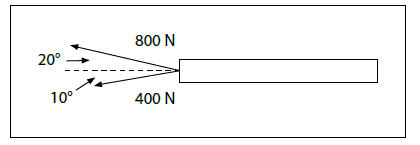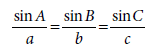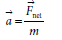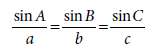# Forces 2 dimensions: How far have the tugboats moved the log

• Evangeline101

## Homework Statement

Two tugboats are pulling on a large log, as shown in the following diagram. The log has a mass of 250 kg and is initially at rest. How far have the tugboats moved the log after 10 s?## Homework Equations

c2 = a2 + b2 - 2abcosC

c = (a2 + b2- 2abcosC)1/2## The Attempt at a Solution

[/B]
c2 = a2 + b2 - 2abcosC

c = (a2 + b2- 2abcosC)1/2

c = [(400 N)2 + (800 N)2 - 2 (400 N) x (800 N) cos 150°]1/2

c= 1164 N

sinβ/800 N = sin 150°/ 1164 N

β = 20°

(To determine the angle for Fnet, subtract 10° from 20°, which equals 10°, as this results in the angle needed to describe the direction for Fnet.)

Fnet = 1164 N [W 10° N]

a = 1164 N [W 10° N] / 250 kg

= 4.66 m/s2 [W 10° N]

Δd = ½ 4.66 m/s2[W 10° N] x (10 s)2

Δd = 233 m [W 10° N]

The tugboats have moved the log 233 m [W 10° N] after 10 seconds.

Is this correct?

## Homework Statement

Two tugboats are pulling on a large log, as shown in the following diagram. The log has a mass of 250 kg and is initially at rest. How far have the tugboats moved the log after 10 s?
View attachment 100372

## Homework Equations

c2 = a2 + b2 - 2abcosC

c = (a2 + b2- 2abcosC)1/2View attachment 100373

## The Attempt at a Solution

[/B]
c2 = a2 + b2 - 2abcosC

c = (a2 + b2- 2abcosC)1/2

c = [(400 N)2 + (800 N)2 - 2 (400 N) x (800 N) cos 150°]1/2

c= 1164 N

sinβ/800 N = sin 150°/ 1164 N

β = 20°

(To determine the angle for Fnet, subtract 10° from 20°, which equals 10°, as this results in the angle needed to describe the direction for Fnet.)

Fnet = 1164 N [W 10° N]

a = 1164 N [W 10° N] / 250 kg

= 4.66 m/s2 [W 10° N]

Δd = ½ 4.66 m/s2[W 10° N] x (10 s)2

Δd = 233 m [W 10° N]

The tugboats have moved the log 233 m [W 10° N] after 10 seconds.

Is this correct?
Looks good.

Ok thanks :)